• Ref:: Better Explained
• Title:: Math and Analogies
• Year of publication:: 2019
• Category:: Blog
• Topic::
• Related::

Using the symbol 0 to represent nothingness is a really weird concept. And when you've tied your concept of a number to physical items, concepts like zero become difficult. How about this negative number? What's less than nothing? What's a negative rock?

It took a few thousand years to accept this new feature — negative numbers were still controversial in the 1700s! Negatives aren’t something we can touch or hold, but they describe certain relationships well (like debt). It was a useful fiction.

An idea: numbers are 2D directions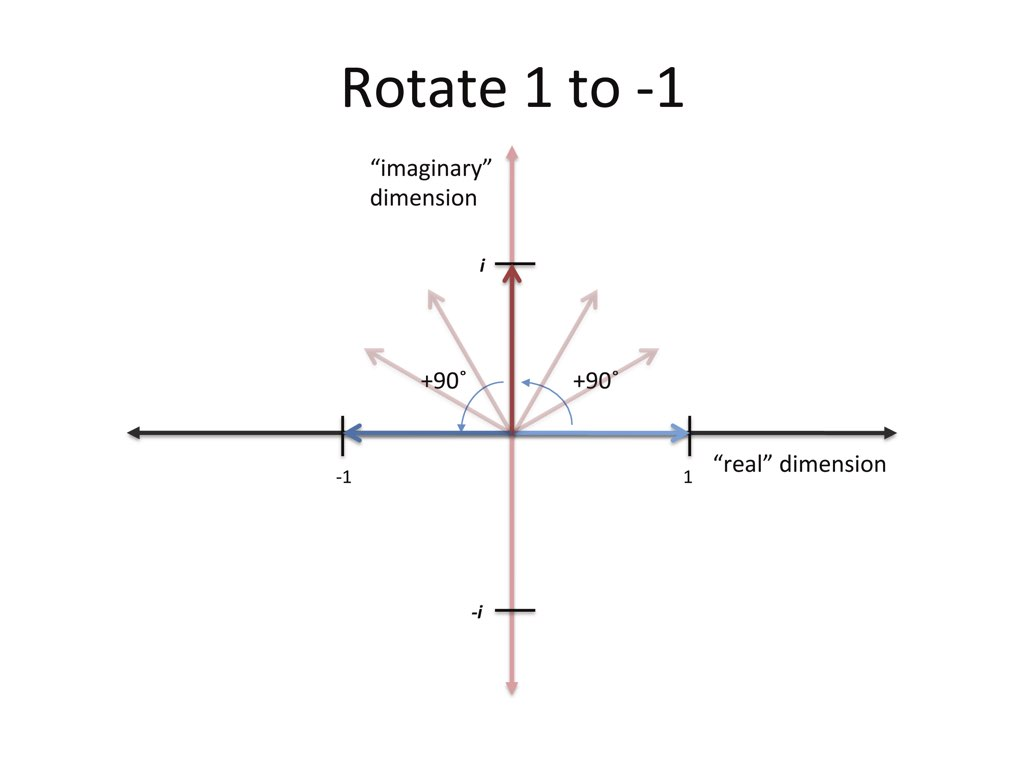The imaginary dimension lets us get to negatives in two steps. Saying "i squared is negative one" is really saying: I'm starting at 1. We multiply by i, multiply by i again, and get to -1. So i represents a 90-degree rotation, and rotating twice points you backwards. That's what "i squared equals -1" means.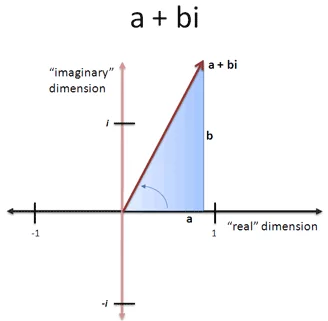what multiplication of two complex numbers does:

• Regular multiplication ("times 2") scales up a number (makes it larger or smaller)
• Imaginary multiplication ("times i") rotates you by 90 degrees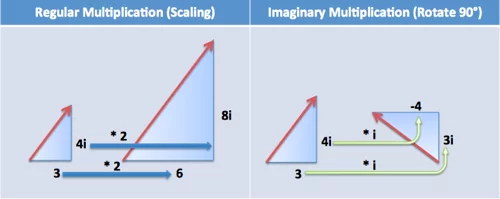Multiplying by (2 + i) means double your number and add in a perpendicular rotation

What about visualizing the multiplication of two complex numbers ("triangles"), like $(3+4i).(2+3i)$. I see this as: Make a scaled version of our original triangle (times 2) and add a scaled/rotated triangle (times 3i)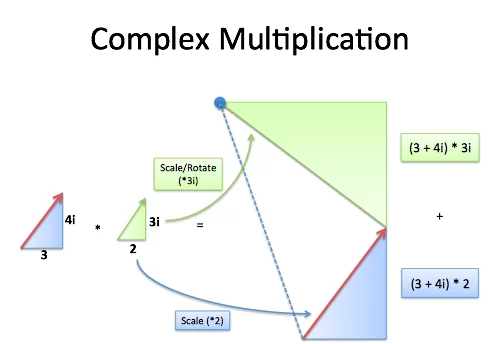Let’s take a look. Suppose I’m on a boat, with a heading of 3 units East for every 4 units North. I want to change my heading 45 degrees counter-clockwise. What’s the new heading?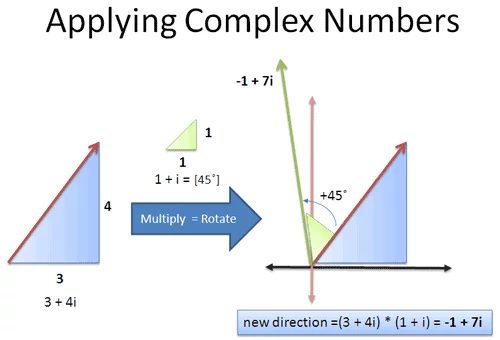An approach by complex number:

• We’re on a heading: 3 units East, 4 units North = $3 + 4i$
• we want to rotate counter-clockwise by 45 degrees = multiply by $1 + i$
• We noticed that 45 degrees is $1 + i$ (perfect diagonal), so we can multiply by that amount

By multiplying together: $(3+4i).(1+i)=3+3i+4i+4i^2=-1+7i$

So our new orientation is 1 unit West (-1 East), and 7 units North